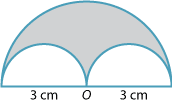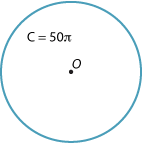#### Example 1

Find the circumference of a circle:
1. whose diameter is 14 cm
2. whose radius is 21 cm.
1. in terms of π
2. as an approximate value, using π ≈ $$\dfrac{22}{7}$$
3. as an approximate value using π ≈ 3.14.

#### Solution

 \begin{align}{\bf \text{a i}}\hspace{20px} C &= \pi d\\\\&= 14\pi\ \text{cm}\end{align} \begin{align}{\bf \text{a ii}}\hspace{15px} C &= 14\pi\\\\&\approx 14 ×\dfrac{22}{7}\\\\&= 44\ \text{cm}\\\\\end{align} \begin{align}{\bf \text{a iii}}\hspace{10px} C &= 14\pi\\\\&\approx 14 × 3.14\\\\&= 43.96\ \text{cm}\end{align} \begin{align}{\bf \text{b i}}\hspace{20px} C &= 2\pi r\\\\&= 2 × \pi × 21\\\\&= 42\pi\ \text{cm}\end{align} \begin{align}{\bf \text{b ii}}\hspace{15px} C &= 42\pi\\\\&\approx 42 ×\dfrac{22}{7}\\\\&= 132\ \text{cm}\end{align} \begin{align}{\bf \text{b iii}}\hspace{10px} C &= 42\pi\\\\&\approx 42 × 3.14\\\\&= 131.88\ \text{cm}\end{align}

Note that the answers in parts ii and iii are only approximate, since we have used an approximation for π.

#### Example 2

The figure is obtained by removing the two semicircles from the large semicircle. What is the exact perimeter of the shaded region?Detailed description

#### Solution

This perimeter consists of half the circumference of the large circle (a semicircle) plus two small semicircles, or one whole small circle.

Radius of large circle = 3 cm

Radius of small circles = 1$$\dfrac{1}{2}$$ or $$\dfrac{3}{2}$$ cm

Hence the perimeter = $$\pi × 3 + 2 × \pi × \dfrac{3}{2} = 6\pi$$ cm

#### Example 3

A circle has circumference 50π cm. Find the radius of the circle.#### Solution

\begin{align}\text{Circumference}\ &= 2\pi r\\\\ 2\pi r &= 50\pi\\\\ r &=\dfrac{50\pi}{2\pi}\\\\ &= 25\ \text{cm}\end{align}